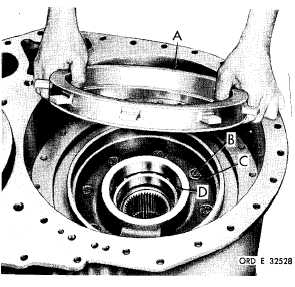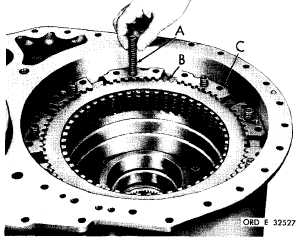Custom Search*C2 P A R    75,   S T E P S   4  3 - 4 6 D I S A S S E M B L Y C H A P   5,   SEC   II Figure   107   (Step   43) Remove   two   bolts   180°   apart,   from   left-output clutch   assembly   (A).   Attach   sling   (B)   and   re- move   left-output   clutch   assembly   (A).   Remove s p a c e r    ( C ). Figure   108   (Step   44) Remove   nine   steer   clutch   release   springs   (A), clutchplates   (B).   Attach   lifting   sling   7083778 at   three   holes   (C)   in   steer   clutch   anchor.   Re- move   the   anchor. Figure   109   (Step   45) R e m o v e    l e f t - s t e e r    c l u t c h    p i s t o n    ( A ) .    U s i n g   a 9 / 1 6 - i n c h     w r e n c h ,     r e m o v e     e i g h t     s e l f - l o c k i ng b o l t s    ( B )    f r o m    o u t p u t    b e a r i n g    r e t a i n e r    ( C ). Remove   retainer   (C)   and   bearing   assembly   (D). Remove   bearing   (D)   from   retainer. Figure   110   (Step   46) R e m o v e    t w o    s c r e w s    ( A )    r e t a i n i n g    r e v e r s e- range   bearing   support   and   carrier   (B). 9 5Integrated Publishing, Inc. - A (SDVOSB) Service Disabled Veteran Owned Small Business